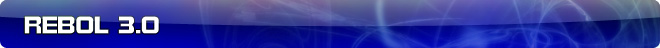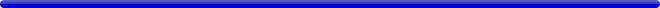# PARSE: the DO keyword

 Carl Sassenrath, CTO REBOL Technologies 16-Oct-2009 0:04 GMT Article #0276

The DO keyword requested by Gabriele has been added to parse in A91.

It is an advanced parse action that evaluates the input block as REBOL code then uses a rule to match the result.

For example:

```>> parse [1 + 2] [do integer!]
== true
```

or, more specifically:

```>> parse [1 + 2] [do quote 3]
== true
```

and:

```>> parse [append "ab" "c"] [do "abc"]
== true
```

and it operates inline, as you can see from the literal word test used below:

```>> parse [test append "ab" "c"] ['test do "abc"]
== true
```

The DO keyword only works with block inputs (not strings), and only specific match cases are valid: skip, none, end, quote, into, and a direct value.

More examples:

```>> parse [] [do end]
== true

>> parse [none] [do none]
== true

>> parse ["abc"] [do none]
== false

>> parse [] [do none]
== false

>> parse ["abc"] [do "abc"]
== true

>> parse [append "ab" "c"] [do "abc"]
== true

>> parse [remove "abc"] [do "bc"]
== true

>> parse ['abc] [do 'abc]
== true

>> parse ['abc] [do 'a]
== false

>> parse ["abc"] [do ["a" "b" "c"]]
== false

>> parse ["abc"] [do string!]
== true

>> parse ["abc"] [do number!]
== false

>> parse ["abc"] [do series!]
== true

>> parse [1 + 2] [do quote 3]
== true

>> parse [1 + 2] [do quote (1 + 2)]
== true

>> parse [append [a] 'b] [do into ['a 'b]]
== true

>> parse [append "ab" "c"] [do into ["ab" "c"]]
== true

>> parse [num: 1 + 2] [
set var set-word!
do [set num integer!]
(print [var num])
]
num: 3
== true
```

Note this final example. Prefix actions are not currently valid for DO. Here, the num is set within the DO match rule. That is, you must write:

```do [set num integer!]
```

not:

```set num do integer!
```

but, that may be added in a future release.

This feature was non-trivial to implement, and it is worth testing well to make sure that it's solid.